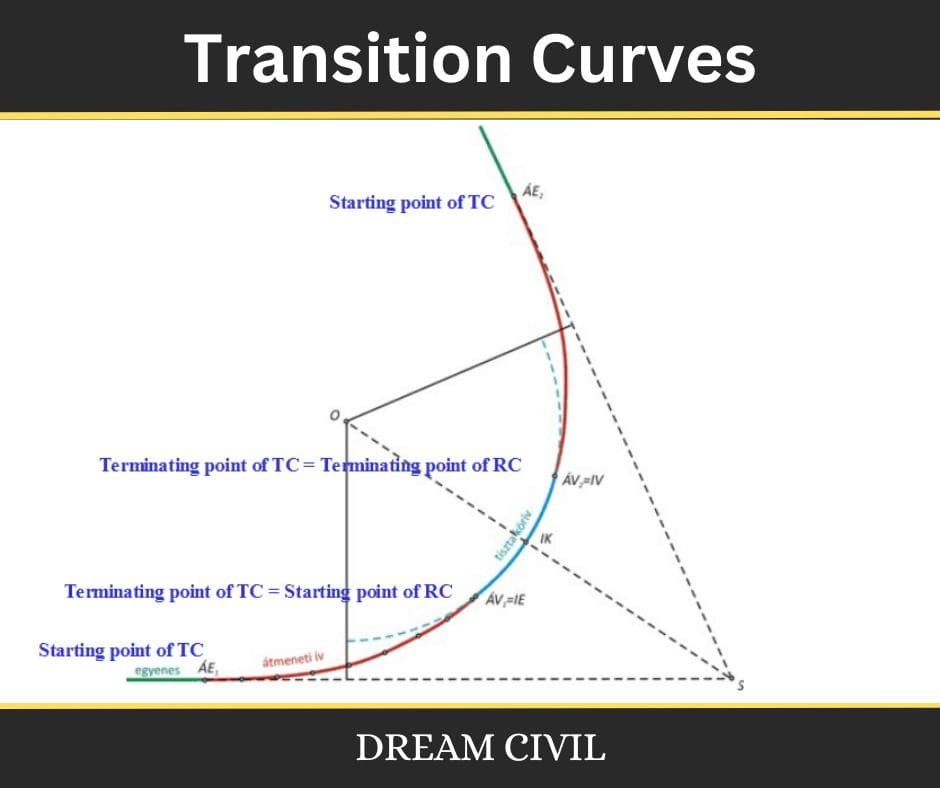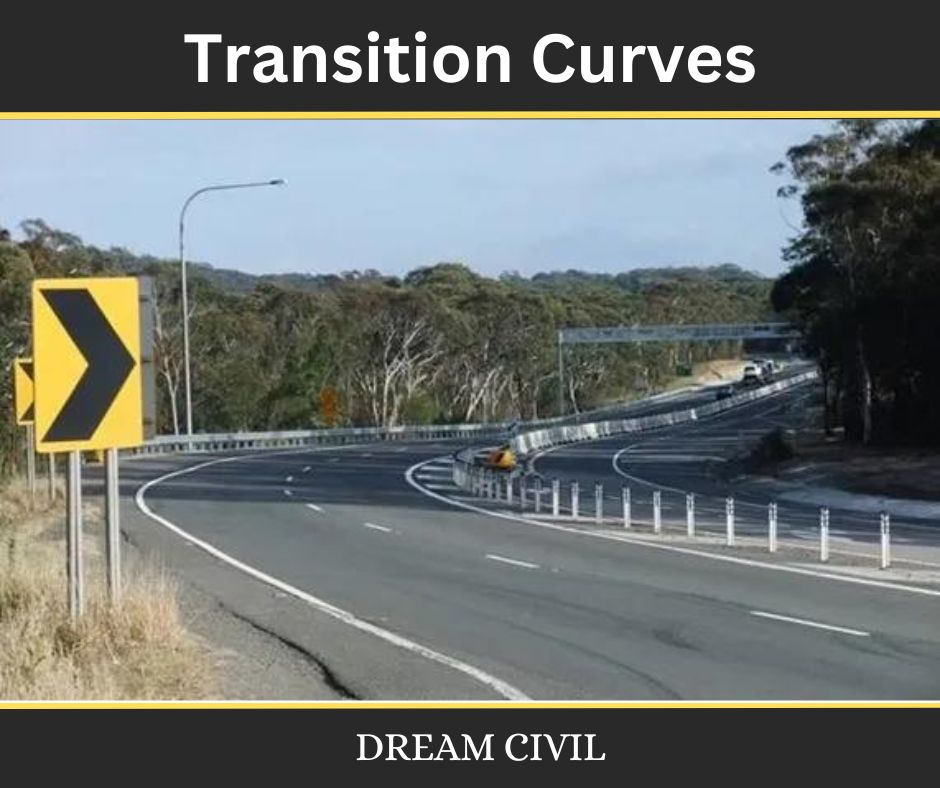Wednesday, October 4, 2023

# Transition Curves : Function, Condition of Transition Curves in Roads

A Transition curve or Easement curve is a curve of varying radius introduced between a Straight and a circular curve or between two branches of a compound curve or reverse curve.

In the case of a highway, in order to hold the vehicle in the center of the lane, the driver is required to move his steering almost instantly to the position necessary for the curve at the moment he passes the P.C.In doing so, the sudden impact of centrifugal force coupled with the inertia of the vehicle would cause the vehicle to sway outwards, and if this exceeds a certain value the vehicle may overturn.

In the case of railways, the side thrust is wholly taken by the pressure exerted by the rails on the flanges of the wheels thus causing wear of the rail in the region of the tangent point.

To avoid these effects, a curve of changing radius must be introduced between the straight and the circular curve.

### 2. Functions of a Transition Curve

The functions of a transition curve are:

a. To accomplish gradually the transition from the tangent to the circular curve, so that the curvature is increased gradually from zero to a specified value.

b. To provide a medium for the gradual introduction or change of the required super-elevation.

### 3. Condition For Transition Curves

A transition curve introduced between the tangent and the circular curve should fulfill the following conditions:

a. It should be tangential to the straight.

b. It should meet the circular curve tangentially.

c. Its curvature should be zero at the origin on straight.

d. Its curvature at the junction with the circular curve should be the same as that of the circular curve.

e. The rate of increase of curvature along the transition should be the same as that of increase of cant or super-elevation.

f. Its length should be such that full cant or super-elevation is attained at the junction with the circular curve.### 4. Objectives for Providing Transition Curve

The objectives of providing Transition curves are mentioned below:

a. For the gradual introduction of Centrifugal force.

b. To introduce super elevation gradually.

c. To introduce extra widening gradually.

d. To provide comfort for the driver that is to enable smooth vehicle operation on the road.

e. To enhance the aesthetics of highways.

### 5. Transition Curve Length Calculation

The length of the transition curve is a necessary factor as it instantly impacts the rate of accomplishment of centrifugal force. The length of a transition curve is inversely proportional to the radius of the horizontal curve. There are three manners by which a transition curve’s length can be selected as mentioned below.

#### 1. Rate of Change of Centrifugal Acceleration

This plan relies on the allowable rate of change of centrifugal acceleration. The centrifugal acceleration is defined as, (V^2)/R. The rate of change of centrifugal force is given as,

c = (V^2)/(R*t)

where

t = V/L (length of transition curves)

Therefore, Lenght of transition curve (L) = (V^3)/(c*R)

c is empirically given as c = 80/(75 + v)

where,

c is in m/(s^3)

v is design speed in Kmph

c is usually taken between 0.5 to 0.8 m/(s^3)

#### 2. Rate of Attainment of Superelevation

This plan relies on the rate of attainment of superelevation. There are two subdivisions within this process as mentioned below.

a. Inner edge rotation

Length of transition curve (L) = N*e*B

where,

N – the rate of change of superelevation (usually 150)

e – superelevation rate

B – width of carriage including widening

b. Centre line rotation

Lenght of transition curve (L) = (N*e*B)/2

where,

N – the rate of change of superelevation (usually 150)

e – superelevation rate

B – width of carriage including widening

#### 3. Empirical Formula

An empirical method established on road terrain is mentioned in the table below.

 Terrain Cross slope Length of transition curve Plain 0 to 10% 2.7*(V^2)/R Rolling 10 to 25% 2.7*(V^2)/R Mountain 25 to 60% (V^2)/R Steep >60% (V^2)/R

### 6. References

1. Content Filter & Authenticity Checking Team, Dream Civil International

(Our team checks every content & detail to maintain quality.)He is a founder and lead author of Dream Civil International and his civil engineering research articles has been taken as source by world's top news and educational sites like USA Today, Time, The richest, Wikipedia, etc.
Latest Articles

Related Articles